# Machine Learning Summary (Proof & Terms)

### Terms

A random variable is a variable holding an outcome in a random process e.g. the observed value in rolling a die. We consider its value is generated by some data distribution (say P(x=1)=P(2)=P(3)=P(4)=P(5)=P(6)=1/6).

Stochastic process or random process contains sequences of events with values determined by probabilities. Technically, it is modeled by an indexed collection of random variables. (usually time-indexed, like Xt).

The Strong law of large numbers states that the average of the results from a large number of trails will be close to the expected value and its difference will get smaller and smaller with more trails.

Conjugate prior: If the posterior distributions calculated by the Bayes’ theorem are in the same distribution family (say Gaussian distribution) as the prior, the prior is a conjugate prior for the likelihood function. Conjugate prior opens the possibilities of calculating the distribution for the Bayes’ Theorem result analytically.

Parametric model vs non-parametric model: A parametric model captures a model with equations and parameters. We use the training data to estimate the model parameters. We don’t need the training data when making an inference. A non-parametric model uses the similarity between training data and the testing data to make a prediction. The K-nearest neighbors classifier is a typical non-parametric model.

Bayes’ Theorem terms:

With the exception of the marginal probability, posterior, prior and likelihood are not a point estimate. It is a curve. In the example below, they show the probability density function (PDF) on different car locations.

Marginal probability normalizes the posterior to be between 0 and 1. Here are the explanations on different terms. In this example, we want to find the infection rate for flu in the current season.

Maxwell–Boltzmann distribution

The probability density function of a random variable at temperature T.

Autoencoder

Autoencoder composes of an encoder to discover latent factors and a decoder to reconstruct the input. By minimizing the reconstruction error, we create a model to extract latent factors and to reconstruct from it.

One-hot vector

Encode categorical information.

Overfit

Precision, recall & F1 score

Measurement metrics:

Gradient descent optimization methods (Momentum, Adagrad, RMSprop, Adam)

Advanced optimization methods based on gradient descent.

Autoregression

Use the previous timestep state(s) as input(s) to solve a regression problem.

Gram matrices

Box plot & Scatter plot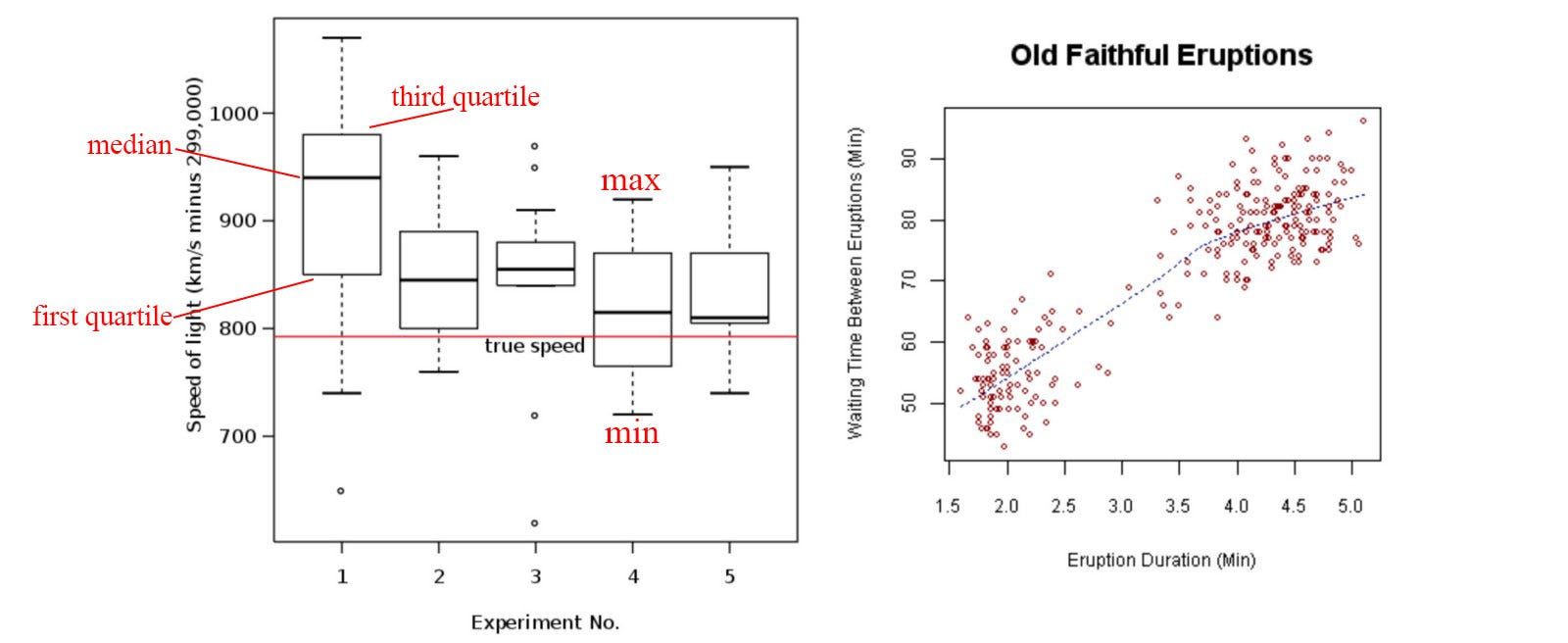Modified from Wikipedia

Exploratory data analysis

Understand the data insights and relationship through visualization or statistical analysis. The following is a Multivariate analysis which each box varies two variables at a time.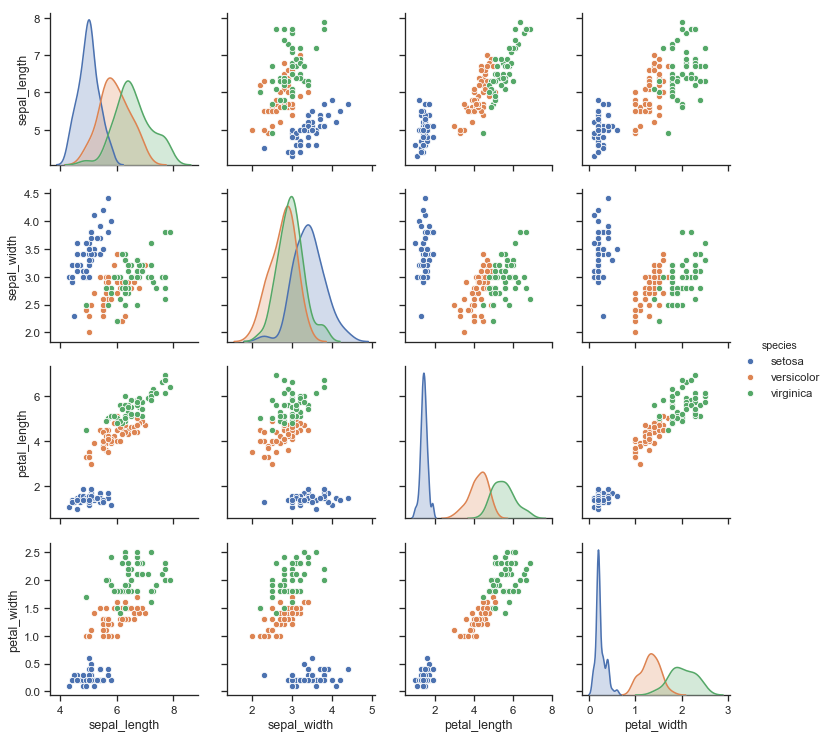Source

Categorical Variable

A variable contains discrete values, e.g. (apple, orange, …, peach)

Lasso Regression

Lasso regression is a linear regression using L1 regularization and squared errors.

Activation function

Sigmoid, ReLU, leaky ReLU and tanh in creating non-linearity of a model:

Bucketing

Encode a continuous variable with buckets.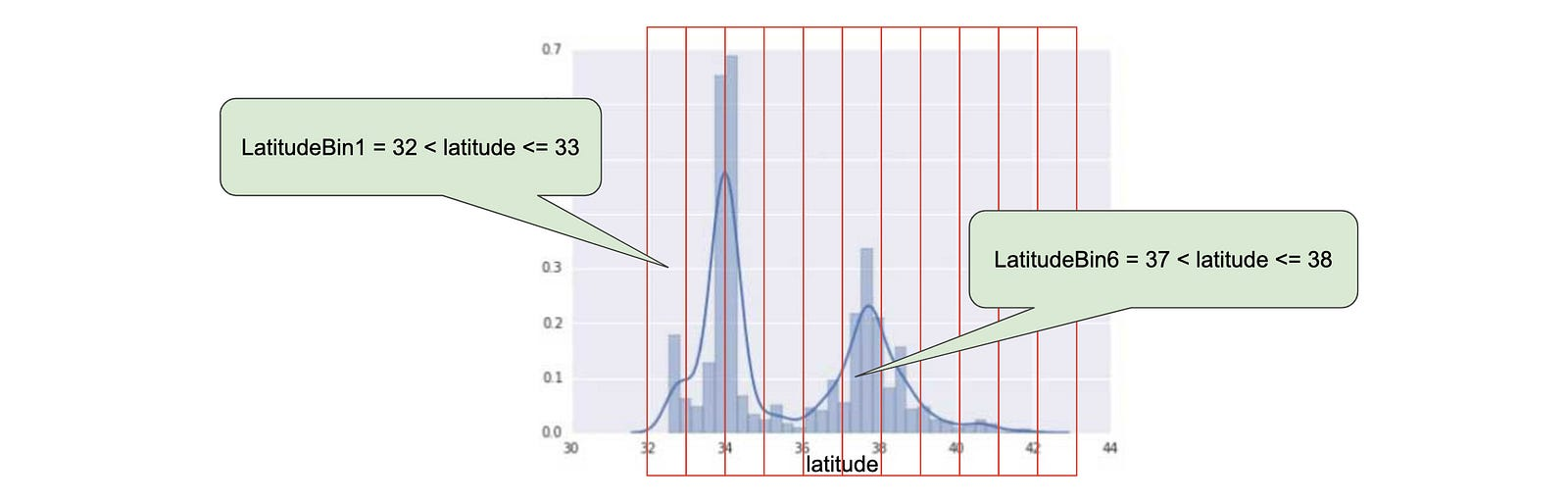Source

AUC (Area under curve)

Find the area under a curve.

Spectral theorem

Every n × n symmetric matrix S has n real eigenvalues λᵢ with n chosen orthonormal eigenvectors vᵢ.

These eigenvalues can form a diagonal matrix Λ as diag(λ). We can also concatenate eigenvectors vᵢ to form V. i.e.,

We rename V as Q. Because Q is orthogonal, it is invertible and Qᵀ = Q⁻ ¹. Therefore, the symmetric matrix S can be factorized into

This is the Spectral theorem on how to diagonalized a symmetric matrix.

Confusion matrix

A confusion matrix holds the values of true positive, false positive, true negative and false negative.Source

independently and identically distributed i.i.d.

Data points are drawn from a distribution that doesn’t change, and each drawn value does not depend on others.

logits

The ratio p/(1-p) is called odds. logits (log odds) takes the log of odds.

Covariates, measurement, features, inputs, independent variables, dependent variable, response, output, prediction

We call the input x to a model f(x) covariates, measurement, features, inputs, or independent variables. We call the output dependent variable, response, prediction or output.

Covariate shift

For example, we train a model for the housing price in San Francisco to predict the housing price for Beverley Hill. The predictions will not be accurate due to the covariate shift. (a.k.a. a shift in the data distribution between the data in training and inference.)

Generative classifier & Gaussian Discriminant Analysis

Cross-validation

We divide the sample into K groups. We use K-1 groups to train the model and one to collect validation performance. We rotate that held-out group for k times and aggregate the performance results from the hold-out groups to access how good the model is. In particular, how good the model can be generalized and not overfitted. We use this method to evaluate the gap between the training error and the validation error. Or we can use that to select hyperparameters. Nevertheless, it is less popular in DL when we prefer to collect a larger dataset and use some part of it dedicated to validation and testing.

Perceptron algorithm

Perceptron uses the gradient descent method to learn a classifier assuming all data points are linearly separable into 2 classes.

Here are the cost function and gradient.

Convexity

Inside a shape, if it is convex, any points between two points fall inside the shape also.

Primal and dual problems

Primal problem: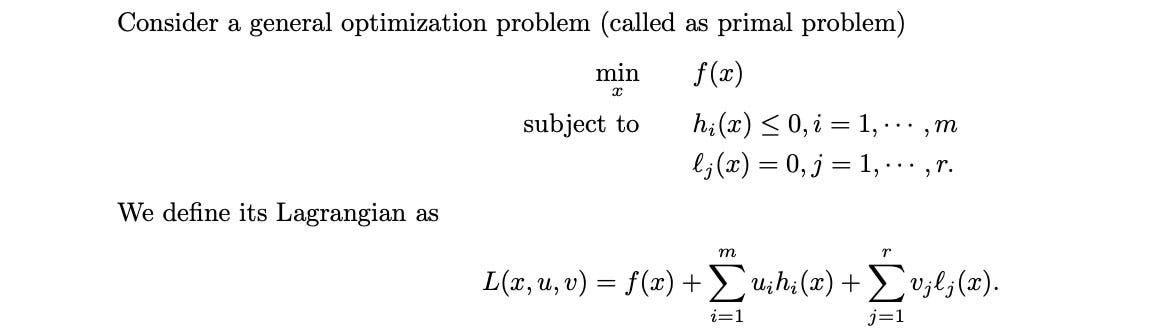Source

Lagrange dual problem:

Hard clustering & soft clustering

In clustering, the use of a probability in evaluating the cluster assignment for each data point is called the soft clustering. Alternatively, we can assign a data point to a cluster deterministically in each training iteration. This is called hard clustering. Soft clustering usually has a smoother cost function that makes training easier and more stable.

Jensen’s inequality

For a convex function, Jensen’s inequality is the inequality underlined in red below.

For a concave function, the inequality will be

Sample space, Event space

The sample space Ω for rolling dice is {1, 2, 3, 4, 5, 6}. p(ω) is the probability for each outcome. For a fair dice, p(ω=1) = 1/6.

Set of events (event space) is F = {{1}, {2}, …, {1, 2}, {2, 3}, …, {1, 2, 3}, … , {1, 4, 7}, …}. Eeah element A in F is called an event. It is is a subset of the outcomes Ω (A⊆Ω). For example, P(A) = 3/6 for the event A {1, 3, 5} which the dice value is odd.

### NLP

Bag of words

Bag of words counts the frequency of words without preserving the ordering.

n-gram & Bigram

n-gram is a contiguous sequence of n items from a given text (an ordered sequence of n words.) and bigram is a 2-gram.

Skip-gram model and Continuous Bag-of-Words model (CBOW)

Skip-gram model predicts each context word from its target word. CBOW is the opposite which we predict the target word from its context.

Negative sampling

Part-of-speech (POS) tagging

Named Entity Recognition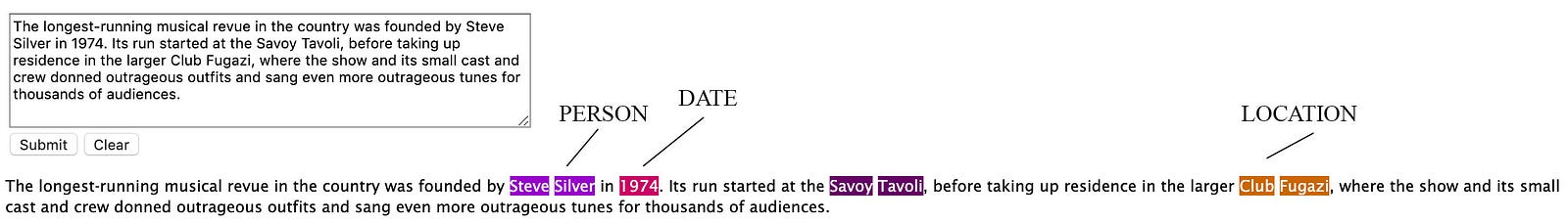Source

### Proof

Optimal model parameters for linear regression with MSE

Sample variance

If data x is Gaussian distributed with limited samples, the tradition equation for variance is a biased estimation for σ².

To correct the bias, we introduce a new estimator for the variance, the sample variance:

Variance in estimating Bernoulli Distribution

Poisson Distribution

Logistic loss

In logistic regression, we compute the probability of being a class Y as

Gaussian process with Cholesky decomposition

#### Probability ratio for logistic regression

Logistic regression

This is the Hinge loss and we have proven it from the perspective of probability ratio and constraint violation.

Bayes’ theorem

Sometimes the result of the Bayes’ theorem can surprise you. Let’s say there is an uncommon genetic disease that only 0.1% of the population has it. We develop a test of 99% accuracy for the positive and the negative result. i.e. if 100 tests are done on people with the disease, 99 of them will be positive. If 100 tests are done on people without the disease, only 1 will be positive. What is the chance that you have this disease if your test positive? The answer is only 9% which is much smaller than you think. The intuition is that even the test looks accurate, it generates more false positive than true positive because the disease is not common. Therefore, for those tested positive, most of them are false alarms. With Bayes’ theorem, we can demonstrate that it is only 9% of having the disease even if you are tested positive.

Notation: d stands for having the disease and + stands for testing positive. ¬d means not d.

Bayesian inference example

Let’s determine the infection rate (θ) of flu for this season. On the first day of the flu season, we got 10 Flu testing results back. We call this evidence (x). (It is often called observation also.) Our new evidence shows that 4 samples are positive. (x=4 out of 10). We can conclude that (θ=4/10=0.4). Nevertheless, we want to study further by calculating the probability of our evidence given a specific θ, i.e. (p(x|θ) which is called the likelihood of our evidence. Here is the equation.

Here is the plot against θ.

Frequentist makes an inference based on evidence. Bayesian inference combines belief with evidence. If we have collected many sample data, the evidence is strong and the inference will resemble the sampled result. Otherwise, we depend on a prior belief in guiding us before more evidence coming in.

In our example, we had collected 100 months of data on the infection rate for flu. This forms a prior belief on the infectious rate for flu in general — but not the particular strain for this season. In the first iteration, we start with a strong prior based on the past 100 months of information. In the first iteration where new sample data is rare, the posterior will resemble the prior closer.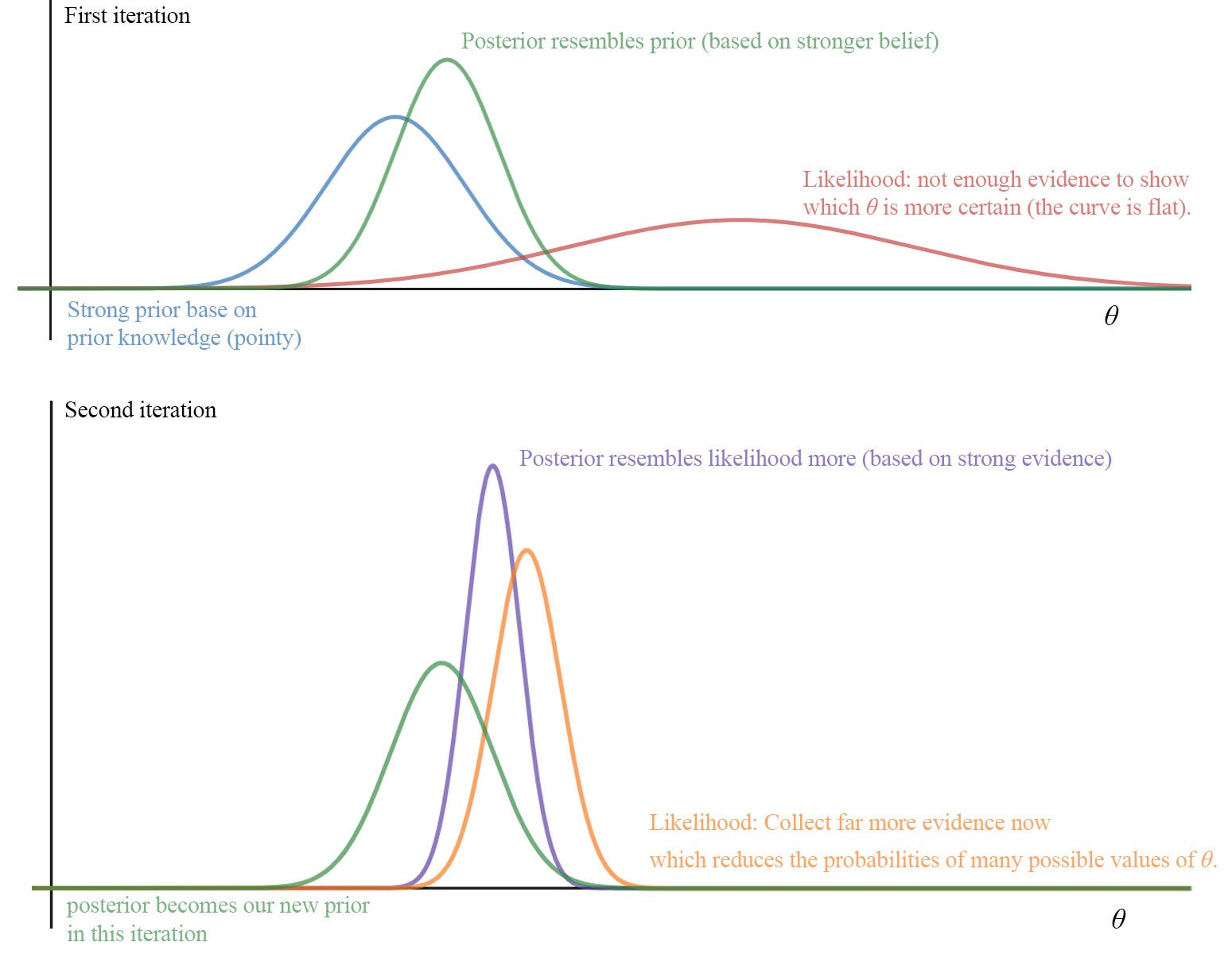illustration purpose only

But as more sample data is collected, the likelihood becomes more certain and the posterior get closer to the likelihood.

Bayes’ Theorem application

There are hidden states of a system that cannot observe directly or easily. Personally, I get some good idea on the chance that I feel happy and sad on average. I also know reasonably well on what I may do when I am happy or sad. On the contrary, the chance of feeling happy given I watch a movie is harder to determine. Here is the information that we collect.

As demonstrated by the Bayes’ Theorem, there is a 94% chance that I feel happy given I went to a party.

HMM

Find the probability of the current state xt given all the observations y.

RBM & Free energy

Free energy F is defined as

Maximize the log-likelihood with the free energy F:

MSE is composed of bias and variance

We are ignoring ε in modeling y = f(x) + ε where ε is the zero-center Gaussian distributed noise with variance σ². If we don’t ignore it, the expected value for the MSE is

Variance

Mean & Variance for MLE estimator

(The proof here utilize the proof in the last section.)

Optimization with constraint(s) — solved by Lagrange multiplier

Minimum L2 Regression

Given

w_in above is a solution for Xw = y. We want to prove that it also satisfies

i.e. w_in has the lowest L2-norm for any solution for y = Xw.

(Proof is originated from here.)

Tower rule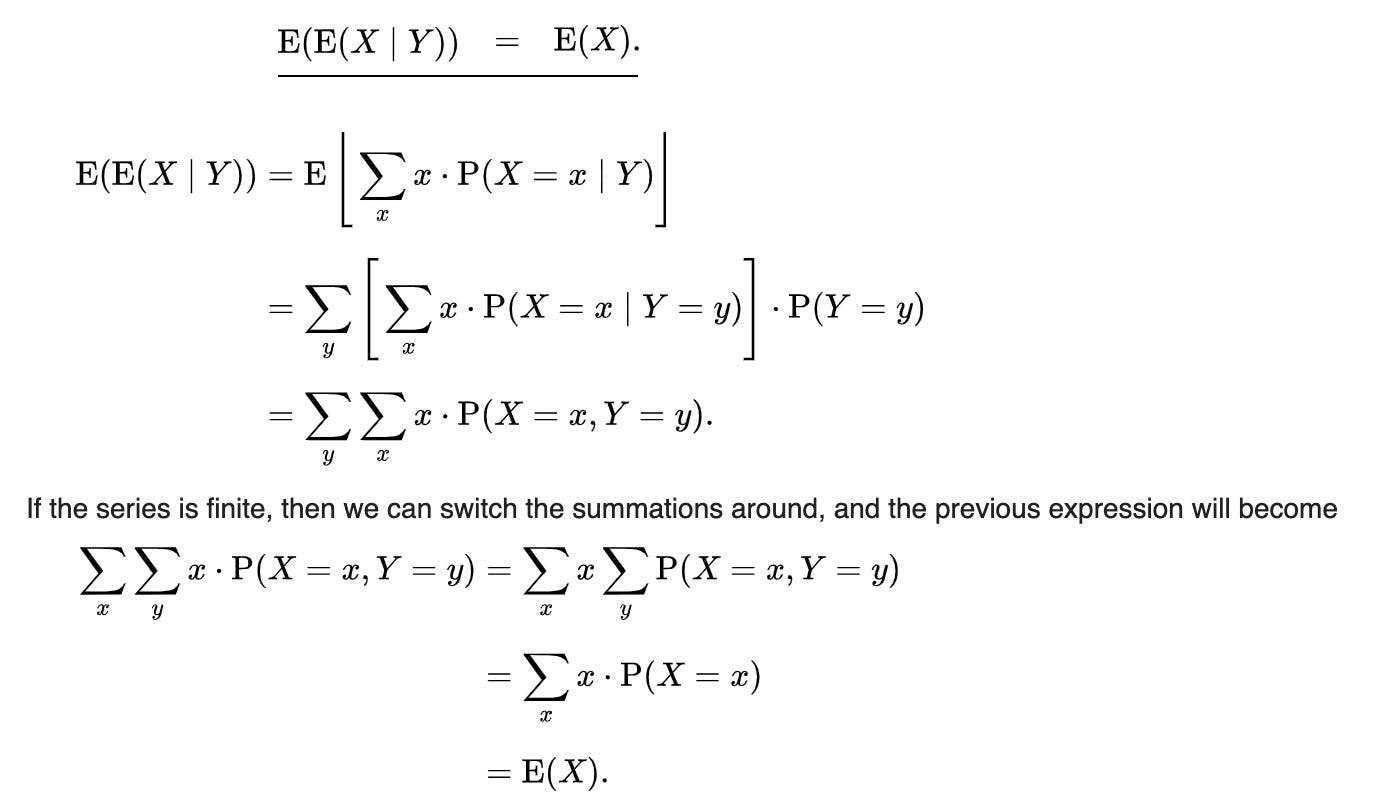Source

c4.5 tree

This algorithm has a few base cases.

• All the samples in the branch belong to the same class. Create a leaf node for it.
• No information gain from any features. C4.5 creates a decision node higher up the tree.

The full algorithm

SVM maximize the margin

Before the proof, we need to understand convexity and the decision boundary first in the next two sections. (For simplicity, we will make claims about convexity and decision boundary without proof here.) Also, the proof here assumes the data points can be completely separated into the corresponding classes.

Convexity representation

Inside a shape, if it is convex, any points between two points fall inside the shape also.

Mathematically, any points inside a convex shape can be represented as a linear combination of other training data points with the conditions below.

Decision hyperplane

The decision hyperplane is always orthogonal to w for linear regression wᵀx.

SVM maximize margin proof

Let’s assume we have two convex shapes that form the boundary of two different classes. For example, the blue pentagon encloses all data points belong to the blue class.

Now, we want to find u and v, the supporting vectors that have the shortest distance among any other possible pairs between these two classes. From the previous section, we show w is always perpendicular to the margin boundary. As shown below, by simple geometry, to have the maximum margin cutting through the supporting vectors, the optimal w* that has the maximum margin ‖ m*‖ must be lying on uv.

Therefore, if we prove the optimal w in the SVM objective is along the line uv, we can prove SVM maximize the margin. (The equations and proof here are originated from Professor Paisley Course.)

Let’s define the supporting vectors first (two shortest distance points between two classes). It can be written as finding point u and v in the following objective using the convexity concept.

The SVM objective is mathematically defined as

We can apply the Lagrange Multiplier to solve the optimization problem with the Lagrangian 𝓛 defined as.

where xᵢ is the training data point i. To optimize the solution, the Lagrange Multiplier involves two steps. First, minimize 𝓛 w.r.t. w and w₀ (the primal problem). We substitute the solution into 𝓛 to create a dual problem that we want to maximize w.r.t. αᵢ. (more information on Lagrange Multiplier)

For the first minimization, we set the corresponding derivative w.r.t. to w and w₀ to 0.

After minimizing the Lagrangian, we apply the solution to 𝓛.

After the substitution, the dual problem (the objective that we want to maximize next) becomes

These can be solved by a software package to find the optimized αᵢ.

After solving αᵢ, we can use condition ① to solve w.

Next, we want to solve w₀. Since our second optimization step maximizes 𝓛 w.r.t. αᵢ, the optimized αᵢ should be greater than 0 only if yᵢ(xᵢᵀw + w₀)-1=0.

So for any αᵢ > 0, we can solve w₀ by solving yᵢ(xᵢᵀw + w₀)-1=0.

Let’s revisit condition ②. The sum of αᵢ (C) from the same class is equal to other class.

We can split the terms below based on the label values which is either +1 or -1 and then divide it by C.

We plug both results into the dual problem.

We get

We realize that maximizing the dual problem is similar to minimizing the term underline in red below.

Notice that this is the same objective in finding the supporting vectors. Let summarize this. We start with the SVM objective function. We use Lagrange Multiplier to solve the problem. It turns out the optimization problem is the same as finding the distance between the supporting vectors — the minimum separation between 2 class boundary. So their optimal solutions fit well with both objectives.

Finally, we need to visualize w and see why SVM maximizes the margin.

i.e. w actual lay on the line uv. Since w is always orthogonal to the decision hyperplane, the corresponding margin boundary will be the maximum. As shown below, if w is not aligned with uv, the corresponding margin will be smaller.

SVM with violation

In practice, due to noise, not all data points are easily separable into the corresponding classes.

We introduce ξᵢ here to have some allowed marginal error for each data point. The SVM objective becomes.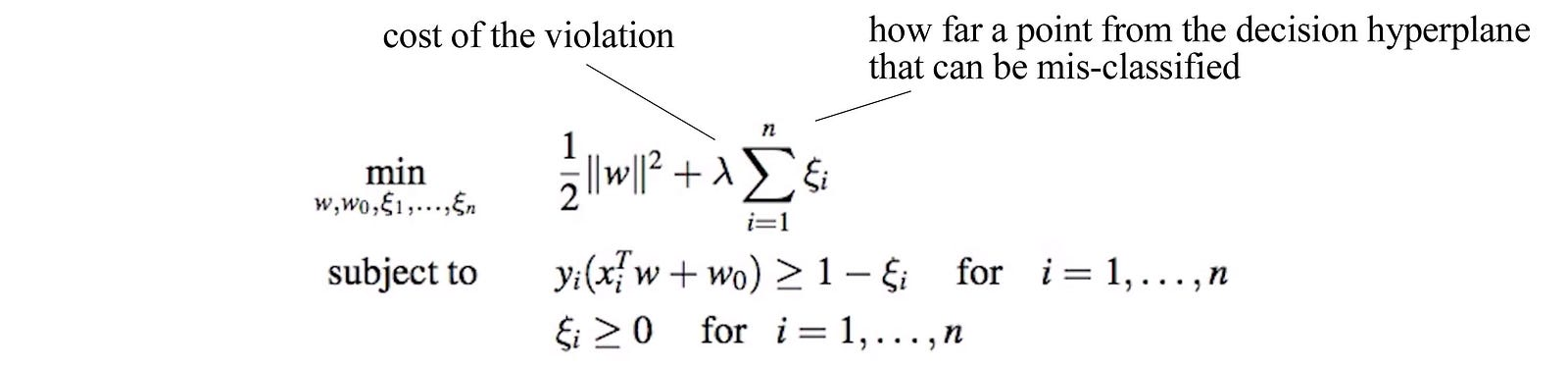Source

The dual problem becomes (it use 𝜙(x) instead of x to expand the concept even further).Source

The optimized solution for the primal problem isSource

Plug in the solution to the Lagrangian. The dual problem that we want to solve becomes (We further expand the concept to include Kernel K)Source

The major difference from our previous solution is the additional constraint αᵢ ≤ λ. Most of the αᵢ will be zero. For αᵢ > 0, those corresponding to the support vectors on the boundary.

To make predictions,Source

AdaBoost error upper bound

(Credit: the equation and the proof is originated from this source).

The upper bound for the training error in AdaBoost is

The proof involves steps to prove each inequality for the condition a ≤ b ≤ c below.

First, let’s review a few equations and definition. In each iteration, we use w(i) to determine the chance of picking data point i. Z is the normalization factor for w (such that all wᵢ add up to one).

We expand the equation for w and define b.

And establish the condition

Next, we want to prove the first inequality a ≤ b.

Next, we want to prove b ≤ c.

Now we can show ac which is what we want to prove.

EM (Proof 1)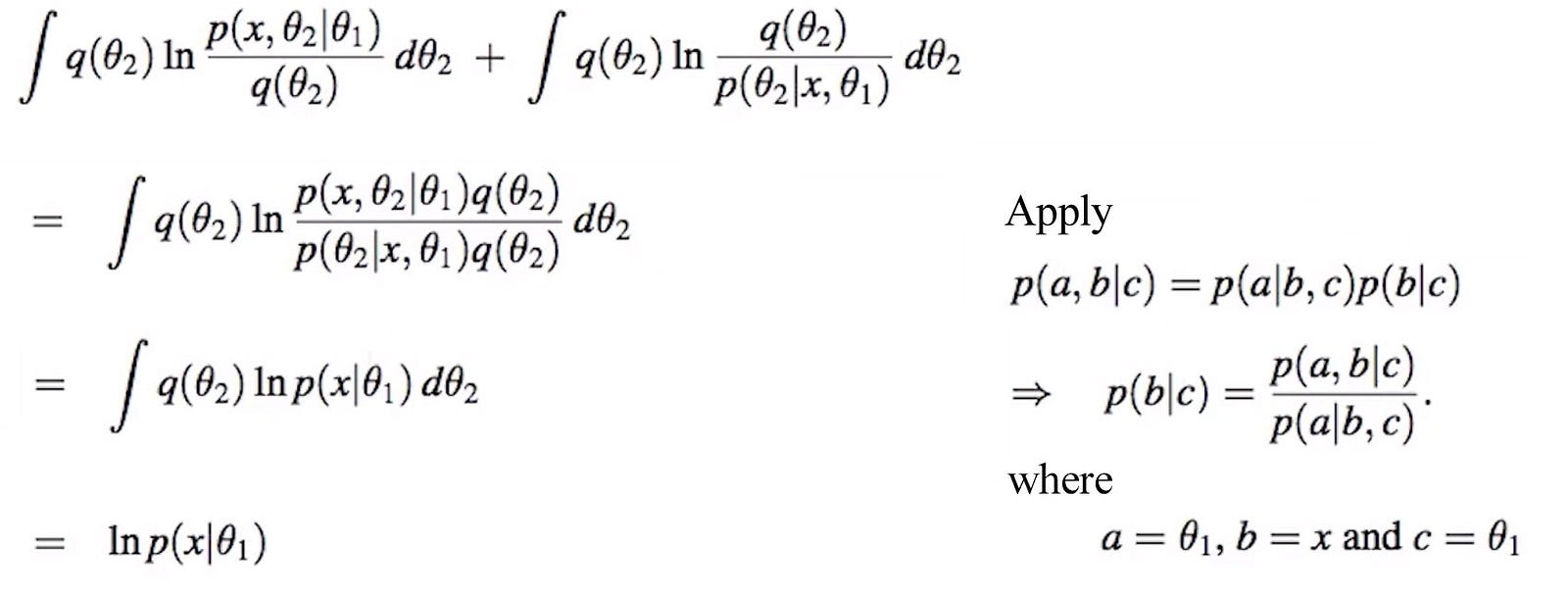Modified from source

EM (Proof 2)

Solving missing data with EM algorithm

(Credit: the equation and the proof is originated from this source).

Consider data xᵢ that have a different number of missing elements at different locations.

We can use the EM algorithm to find the missing data as well as μ and Σ that model x.

In the E-step, we find a method to compute q with p(θ₂|x, θ₁)

Now, we know how to compute q. We can integrate over xᵐᵢ to compute the log-likelihood p(xᵒᵢ | μ , Σ).

We can optimize the log-likelihood in the M-step as

Given the following definitions

The optimized μ and Σ can be computed as:

GMM with EM algorithm

(Credit: the equation and the proof is originated from this source).

Let’s define a model on how data is generated in GMM. For example, say 0.4 chance that the new data belongs to cluster A and 0.6 chance that it belongs to cluster B. Then π = {0.4, 0.6}. Once we have the cluster assignment cᵢ, we sample xᵢ from the corresponding Gaussian distribution of the cluster cᵢ.

We want to learn the model θ₁ with the following parameters.

Let’s start with the log likelihood again in the EM algorithm which is expressed as

and θ₂ is the cluster assignment cᵢ.

Set q(cᵢ = k) to p(cᵢ = k | xᵢ, π, μ, Σ) in the E-step

In the M-step, we cross out the denominator that is constant relative to π, μ, Σ, and the second term which q = p and therefore it is zero.

So the log-likelihood that we want to optimize is:

We can differentiate the objective above w.r.t. π, μ, Σ to find the optimal π, μ, Σ. Here are the solution and the final algorithm.Source

Matrix factorizing

(Credit: the equation and the proof is originated from this source).

Let’s define the notation for the latent factors for user i and item j. Mᵢⱼ contains a valid rating (non-empty entry).

U contains are user latent factors and V contains all item latent factors. The user rating matrix is R = UVᵀ.

We solve it by

But u depends on v and vice versa. So we cannot solve it analytically. But we can solve it in gradient descent in alternating steps.

Here is the final algorithm: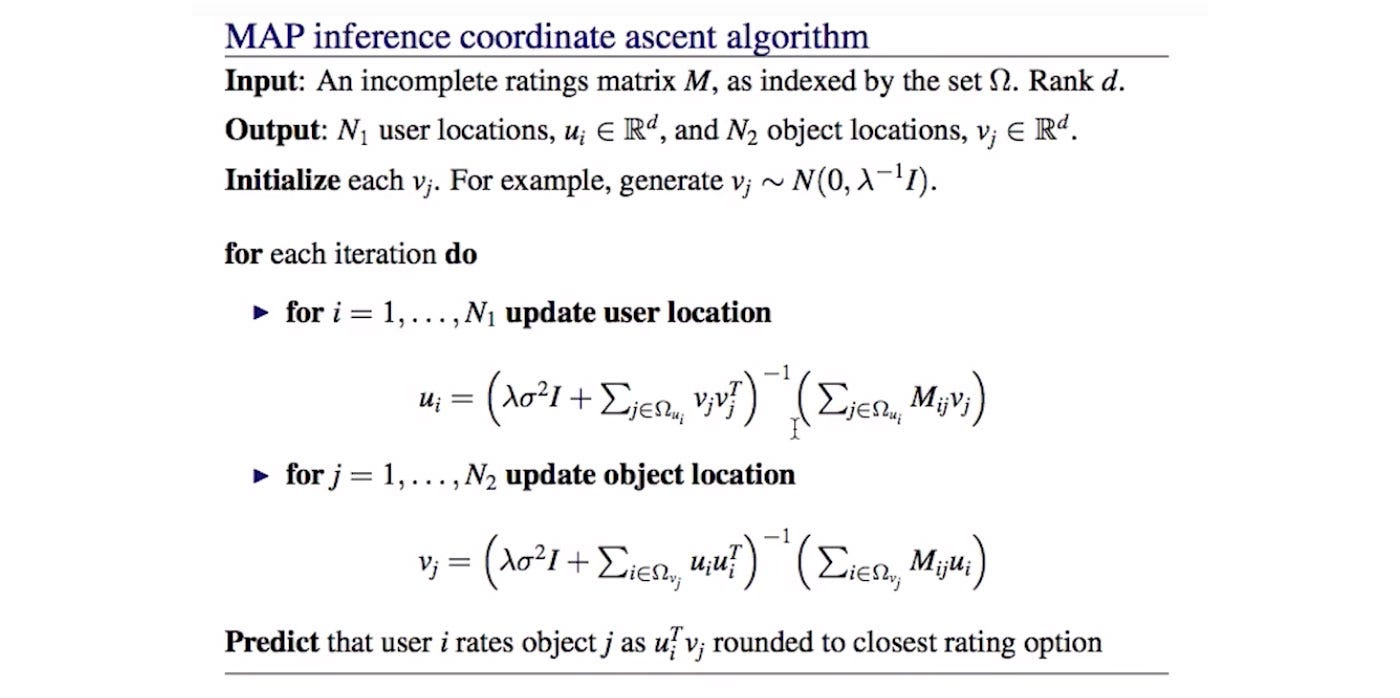Source

PCA with EM algorithm

We model X≈WZ. Here is our generative model for X and z. We are going to learn W and z.

However, it is intractable if we solve it with MLE.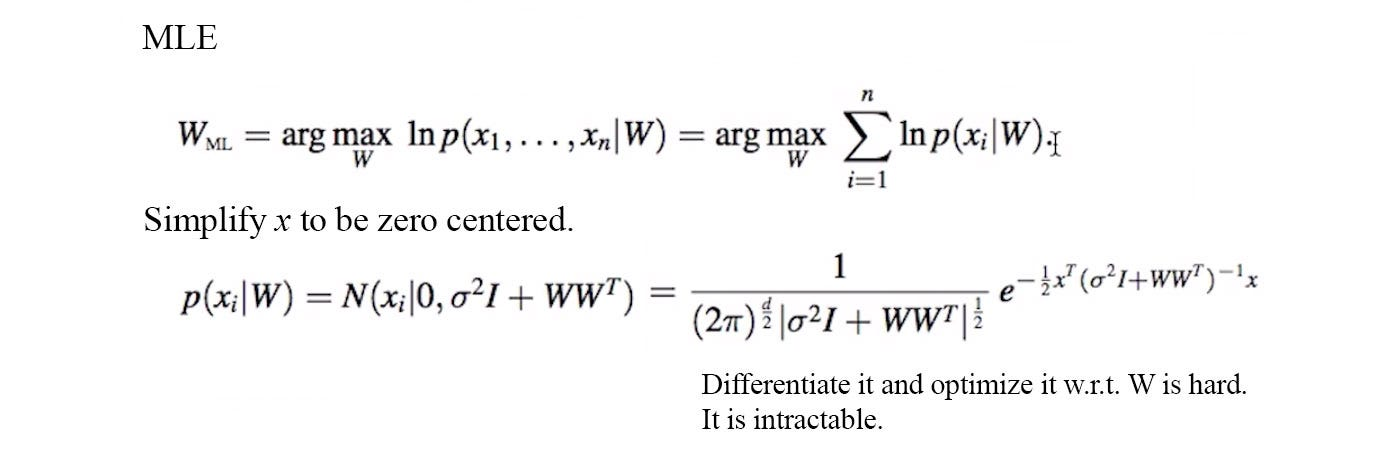Source

Instead, we will apply the EM algorithm.

Without proof, it is the solution.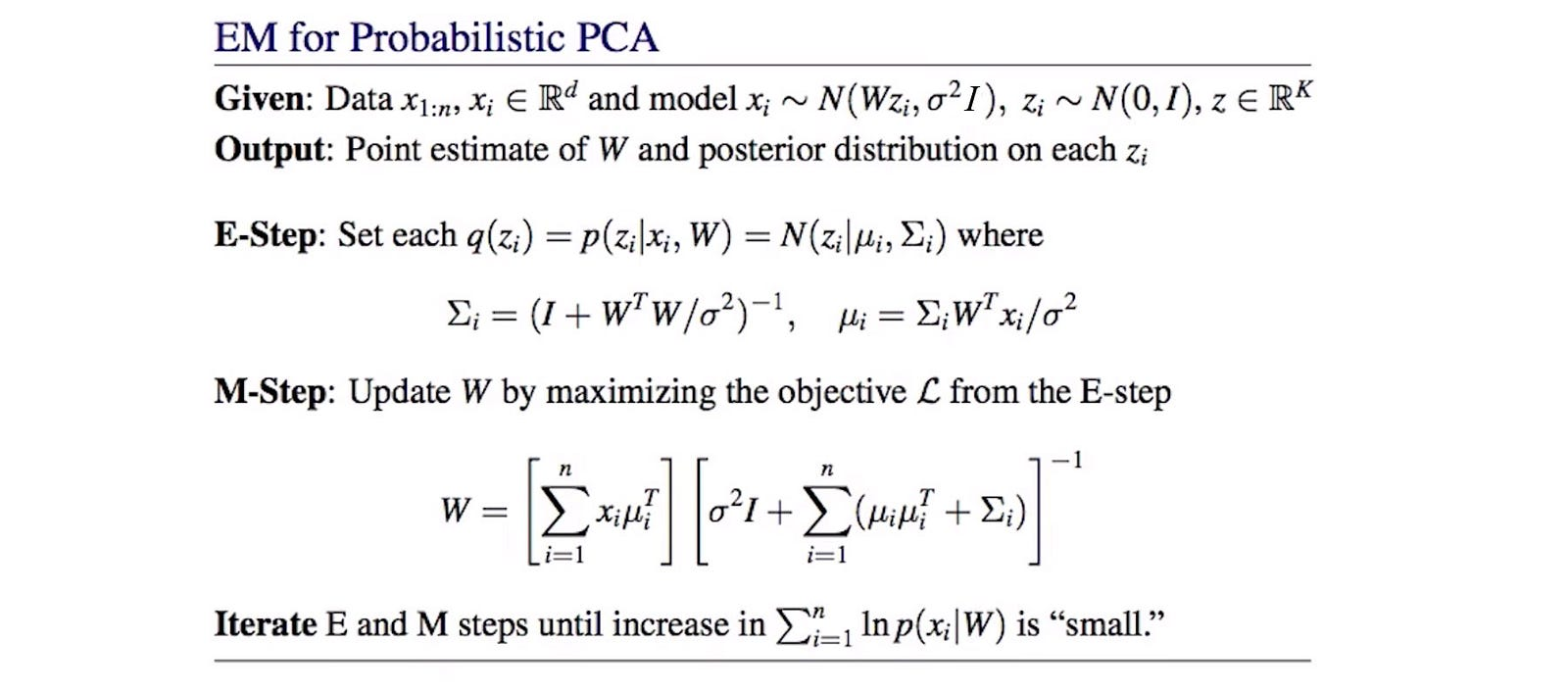Source

Bayesian Information Criterion

(Credit: the equation and the proof is originated from this source).

In BIC, we want to maximize the posterior of the model. After applying the Bayes’ Theorem, we remove any terms that is constant w.r.t. Mᵢ.

The integral above is hard to compute. But we can estimate with the Laplace approximation. Apply Laplace approximation to optimize the objective.

As shown in the discussion of the Laplace approximation. The integral is the normalization factor for the Gaussian distribution.

Recall the following definition with the assumption of i.i.d.:

Plug the value back and we arrive at the BIC penalty.